# 程序员最近都爱上了这个网站  程序员们快来瞅瞅吧！  it98k网:it98k.com

出租广告位,需要合作请联系站长

+关注

2022-02(10)

2022-03(3)

# （一）OpenCV 的 Python 接口

OpenCV 是一个C++ 库，它包含了计算机视觉领域的很多模块。除了 C++ 和 C， Python 作为一种简洁的脚本语言，在 C++ 代码基础上的 Python 接口得到了越来越广泛的支持。

import cv2
1

pip install opencv-python

# （二）OpenCV 基础知识

OpenCV自带读取、写入图像函数以及矩阵操作和数学库。

## （1）读取和写入图像

# -*- coding: utf-8 -*-
import cv2
# 读取图像
h,w = im.shape[:2]
print (h,w)
# 保存图像
cv2.imwrite('alcatraz1.png',im)
12345678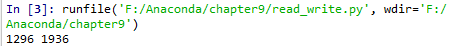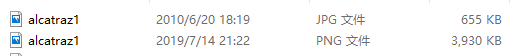# -*- coding: utf-8 -*-

import cv2
# 读取图像
h,w = im.shape[:2]
print (h,w)
# 保存图像
cv2.imwrite('C-uniform01.jpg',im)
123456789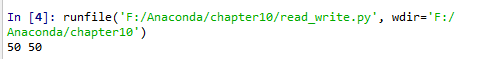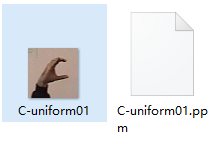## （2）颜色空间

• cv2.COLOR_BGR2GRAY (原图像转化为灰度图像)
• cv2.COLOR_BGR2RGB (原图像转化为RGB图像)
• cv2.COLOR_GRAY2BGR (灰度图像图像转化为BGR图像)

# -*- coding: utf-8 -*-
import cv2
import numpy as np
from pylab import  *
from PIL import Image

# 添加中文字体支持
from matplotlib.font_manager import FontProperties
font = FontProperties(fname=r"c:\windows\fonts\SimSun.ttc", size=14)

# 读取图像
gray = cv2.cvtColor(im1,cv2.COLOR_BGR2GRAY)
BGR = cv2.cvtColor(gray,cv2.COLOR_GRAY2BGR)
figure()
subplot(131)
title(u'(a)原始图像', fontproperties=font)
axis('off')
imshow(im)
subplot(132)
title(u'(b)COLOR_BGR2GRAY转换后图像', fontproperties=font)
axis('off')
imshow(gray)
subplot(133)
title(u'(c)(b)经过COLOR_GRAY2RGB转换后图像', fontproperties=font)
axis('off')
imshow(BGR)
show()
1234567891011121314151617181920212223242526272829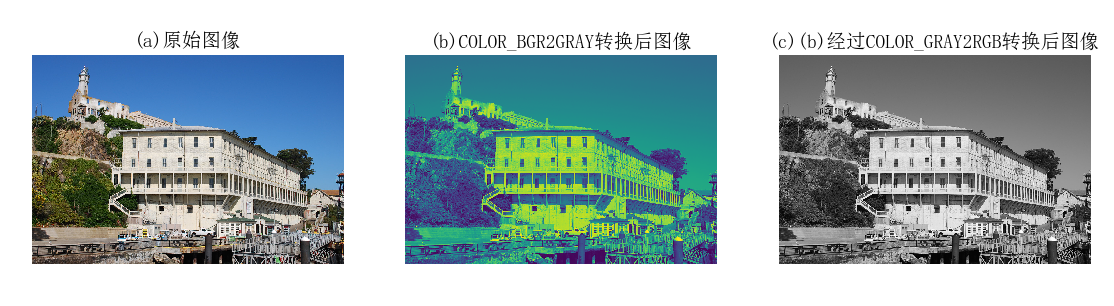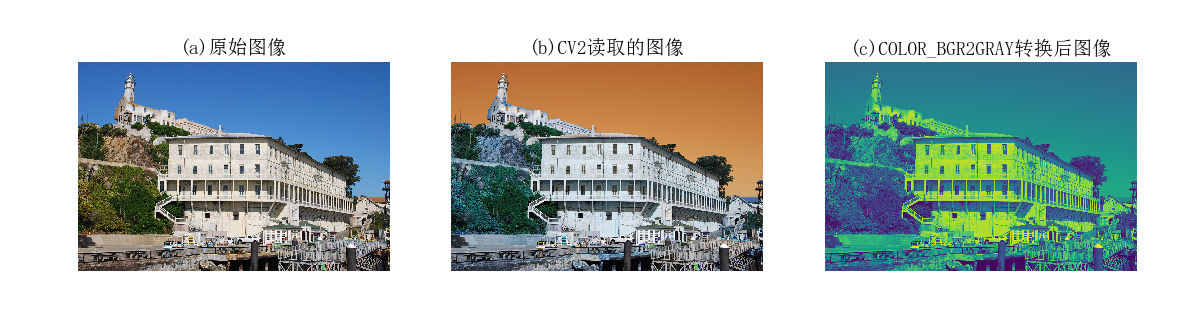## （3） 显示图像及结果

# -*- coding: utf-8 -*-

import cv2
import numpy as np
from pylab import  *
from PIL import Image
# 添加中文字体支持
from matplotlib.font_manager import FontProperties
font = FontProperties(fname=r"c:\windows\fonts\SimSun.ttc", size=14)

# 读取图像
gray = cv2.cvtColor(im,cv2.COLOR_BGR2GRAY)
# 计算积分图像
intim = cv2.integral(gray)
# 归一化并保存
intim = (255.0*intim) / intim.max()

figure()
subplot(121)
title(u'(a)原始图像', fontproperties=font)
axis('off')
imshow(im)
subplot(122)
title(u'(b)归一化后图像', fontproperties=font)
axis('off')
imshow(intim)
show()
12345678910111213141516171819202122232425262728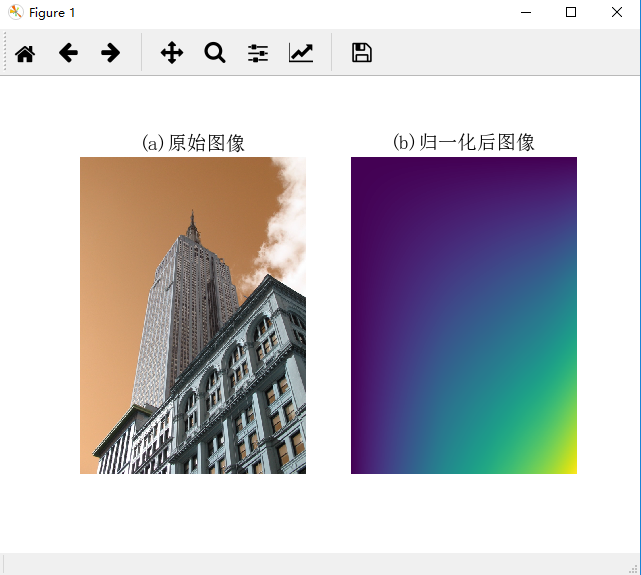# -*- coding: utf-8 -*-
import cv2
import numpy as np
from pylab import  *
from PIL import Image
# 添加中文字体支持
from matplotlib.font_manager import FontProperties
font = FontProperties(fname=r"c:\windows\fonts\SimSun.ttc", size=14)

# 读取图像
h,w = im.shape[:2]

# 泛洪填充
diff = (6,6,6)

# 在OpenCV 窗口中显示结果
cv2.imshow('flood fill',im)
cv2.waitKey()

# 保存结果
cv2.imwrite('result.jpg',im)
123456789101112131415161718192021222324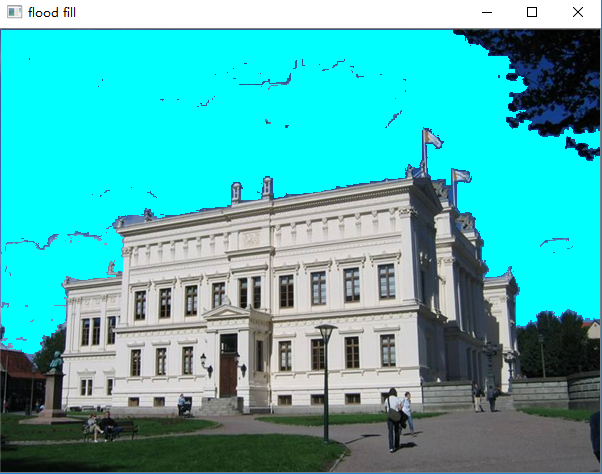# -*- coding: utf-8 -*-
import cv2
import numpy as np
from pylab import  *
from PIL import Image
# 添加中文字体支持
from matplotlib.font_manager import FontProperties
font = FontProperties(fname=r"c:\windows\fonts\SimSun.ttc", size=14)

# 读取图像

# 下采样
im_lowres = cv2.pyrDown(im)

# 变换成灰度图像
gray = cv2.cvtColor(im_lowres,cv2.COLOR_RGB2GRAY)

# 检测特征点
s = cv2.xfeatures2d.SIFT_create()

# 显示结果及特征点
vis = cv2.cvtColor(gray,cv2.COLOR_GRAY2BGR)

for k in keypoints[::10]:
cv2.circle(vis,(int(k.pt),int(k.pt)),2,(0,255,0),-1)
cv2.circle(vis,(int(k.pt),int(k.pt)),int(k.size),(0,255,0),2)
cv2.imshow('local descriptors', vis)
cv2.waitKey()
12345678910111213141516171819202122232425262728293031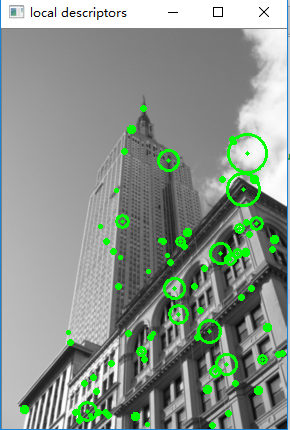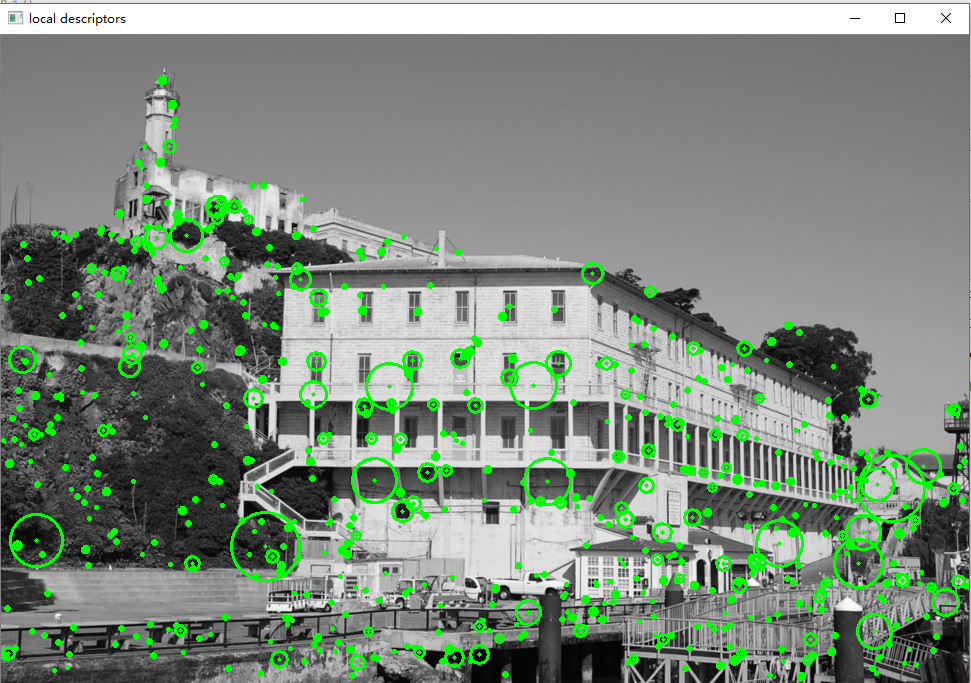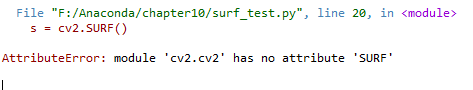# （三）处理视频

## （1）视频输入

OpenCV 能够很好地支持从摄像头读取视频。下面给出了一个捕获视频帧并在 OpenCV 窗口中显示这些视频帧的完整例子，编写实验代码：

# -*- coding: utf-8 -*-
import cv2
# 设置视频捕获
cap = cv2.VideoCapture(0)
while True:
cv2.imshow('video test',im)
key = cv2.waitKey(10)
if key == 27:
break
if key == ord(' '):
cv2.imwrite('vid_result.jpg',im)
123456789101112# -*- coding: utf-8 -*-
import cv2
# 设置视频捕获
cap = cv2.VideoCapture(0)
while True:
blur = cv2.GaussianBlur(im, (0, 0), 5)
cv2.imshow('camera blur', blur)
if cv2.waitKey(10) == 27:
break
12345678910## （2）将视频读取到 NumPy 数组中

# -*- coding: utf-8 -*-
import cv2
import numpy as np
# 设置视频捕获
cap = cv2.VideoCapture(0)
frames = []
# 获取帧，存储到数组中
while True:
cv2.imshow('video',im)
frames.append(im)
if cv2.waitKey(10) == 27:
break
frames = array(frames)
# 检查尺寸
print (im.shape)
print (frames.shape)
1234567891011121314151617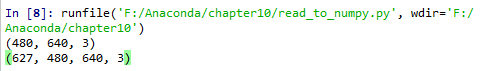# （四）跟踪

## （1）光流

1. 亮度恒定：图像中目标的像素强度在连续帧之间不会发生变化。
2. 时间规律：相邻帧之间的时间足够短，以至于在考虑运行变化时可以忽略它们之 间的差异。该假设用于导出下面的核心方程。
3. 空间一致性：相邻像素具有相似的运动。

$v=[u,v]$是运动矢量，$I_{i}$是时间偏导。对于图像中那些单个的点，该方程是线性方程组。由于 $v$ 包含两个未知变量，所以该方程是不可解的。通过强制加入空间一致性约束，则有可能获得该方程的解。

# -*- coding: utf-8 -*-
import cv2
import numpy as np
from pylab import  *
from PIL import Image

def draw_flow(im,flow,step=16):
""" 在间隔分开的像素采样点处绘制光流 """
h,w = im.shape[:2]
y,x = mgrid[step/2:h:step,step/2:w:step].reshape(2,-1).astype(int)
fx, fy = flow[y, x].T
# 创建线的终点
lines = vstack([x,y,x+fx,y+fy]).T.reshape(-1,2,2)
lines = int32(lines)
# 创建图像并绘制
vis = cv2.cvtColor(im, cv2.COLOR_GRAY2BGR)
for (x1, y1), (x2, y2) in lines:
cv2.line(vis, (x1, y1), (x2, y2), (0, 255, 0), 1)
cv2.circle(vis, (x1, y1), 1, (0, 255, 0), -1)
return vis
#设置视频捕获
cap = cv2.VideoCapture(0)
prev_gray = cv2.cvtColor(im,cv2.COLOR_BGR2GRAY)
while True:
# 获取灰度图像
gray = cv2.cvtColor(im,cv2.COLOR_BGR2GRAY)
# 计算流
flow = cv2.calcOpticalFlowFarneback(prev_gray,gray,None,0.5,3,15,3,5,1.2,0)
prev_gray = gray
# 画出流矢量
cv2.imshow('Optical flow',draw_flow(gray,flow))
if cv2.waitKey(10) == 27:
break
1234567891011121314151617181920212223242526272829303132333435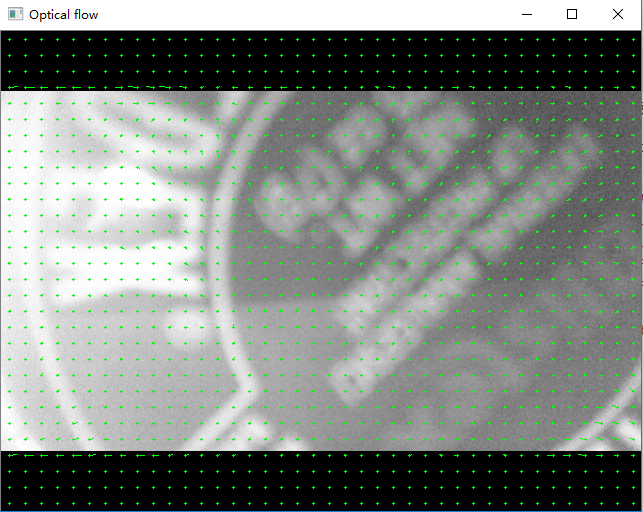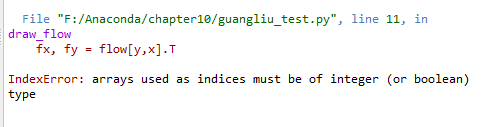y, x = mgrid[step / 2:h:step, step / 2:w:step].reshape(2, -1).astype(int)
#以网格的形式选取二维图像上等间隔的点，这里间隔为16，reshape成2行的array

v 只有在$A^{T}A$ 可逆时才是可解的。这就是 Lucas-Kanade 跟踪算法运行矢量怎样计算出来的全过程。

# -*- coding: utf-8 -*-
from numpy import *
import cv2

# 一些常数及默认参数
lk_params = dict(winSize=(15,15),maxLevel=2,
criteria=(cv2.TERM_CRITERIA_EPS | cv2.TERM_CRITERIA_COUNT,10,0.03))

subpix_params = dict(zeroZone=(-1,-1),winSize=(10,10),
criteria = (cv2.TERM_CRITERIA_COUNT | cv2.TERM_CRITERIA_EPS,20,0.03))

feature_params = dict(maxCorners=500,qualityLevel=0.01,minDistance=10)

class LKTracker(object):

def __init__(self,imnames):
"""  使用图像名称列表初始化 """
self.imnames = imnames
self.features = []
self.tracks = []
self.current_frame = 0

def detect_points(self):
"""  利用子像素精确度在当前帧中检测“利于跟踪的好的特征”( 角点 ) """
# 载入图像并创建灰度图像
self.gray = cv2.cvtColor(self.image,cv2.COLOR_BGR2GRAY)

#  搜索好的特征点
features = cv2.goodFeaturesToTrack(self.gray, **feature_params)

#  提炼角点位置
cv2.cornerSubPix(self.gray,features, **subpix_params)

self.features = features
self.tracks = [[p] for p in features.reshape((-1,2))]

self.prev_gray = self.gray

def track_points(self):
""" 跟踪检测到的特征 """

if self.features != []:
self.step() # 移到下一帧

#  载入图像并创建灰度图像
self.gray = cv2.cvtColor(self.image,cv2.COLOR_BGR2GRAY)

#reshape() 操作，以适应输入格式
tmp = float32(self.features).reshape(-1, 1, 2)

# 计算光流
features,status,track_error = cv2.calcOpticalFlowPyrLK(self.prev_gray,self.gray,tmp,None,**lk_params)

# 去除丢失的点
self.features = [p for (st,p) in zip(status,features) if st]

#  从丢失的点清楚跟踪轨迹
features = array(features).reshape((-1,2))
for i,f in enumerate(features):
self.tracks[i].append(f)
ndx = [i for (i,st) in enumerate(status) if not st]
ndx.reverse() # 从后面移除
for i in ndx:
self.tracks.pop(i)

self.prev_gray = self.gray

def step(self,framenbr=None):
"""   移到下一帧。如果没有给定参数，直接移到下一帧  """
if framenbr is None:
self.current_frame = (self.current_frame + 1) % len(self.imnames)
else:
self.current_frame = framenbr % len(self.imnames)

def track(self):
"""  发生器，用于遍历整个序列 """

for i in range(len(self.imnames)):
if self.features == []:
self.detect_points()
else:
self.track_points()

#创建一份 RGB 副本
f = array(self.features).reshape(-1,2)
im = cv2.cvtColor(self.image,cv2.COLOR_BGR2RGB)
yield im,f

def draw(self):
"""  用 OpenCV 自带的画图函数画出当前图像及跟踪点，按任意键关闭窗口 """

# 用绿色圆圈画出跟踪点
for point in self.features:
cv2.circle(self.image,(int(point),int(point)),3,(0,255,0),-1)

cv2.imshow('LKtrack',self.image)
cv2.waitKey()
123456789101112131415161718192021222324252627282930313233343536373839404142434445464748495051525354555657585960616263646566676869707172737475767778798081828384858687888990919293949596979899100

goodFeaturesToTrack() 方法可以完成这一主要工作。

OpenCV 函数实现了一个完整独立的跟踪系统。

1. 使用跟踪器

# -*- coding: utf-8 -*-
import lktrack
imnames = ['F:\\Anaconda\\chapter10\\images\\bt.005.pgm','F:\\Anaconda\\chapter10\\images\\bt.004.pgm',
'F:\\Anaconda\\chapter10\\images\\bt.003.pgm', 'F:\\Anaconda\\chapter10\\images\\bt.002.pgm',
'F:\\Anaconda\\chapter10\\images\\bt.001.pgm', 'F:\\Anaconda\\chapter10\\images\\bt.000.pgm']
# 创建跟踪对象
lkt = lktrack.LKTracker(imnames)
# 在第一帧进行检测，跟踪剩下的帧
lkt.detect_points()
lkt.draw()
for i in range(len(imnames)-1):
lkt.track_points()
lkt.draw()
12345678910111213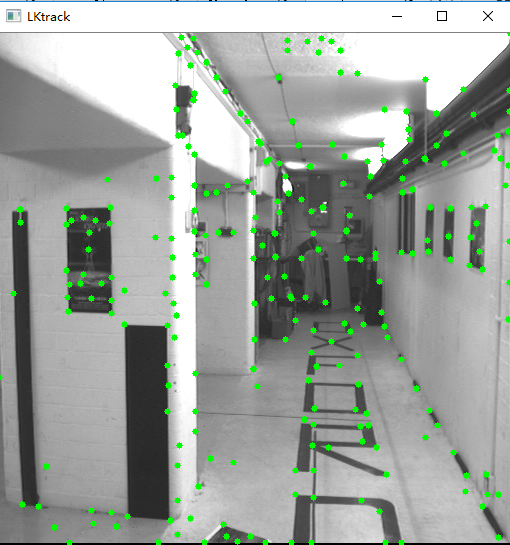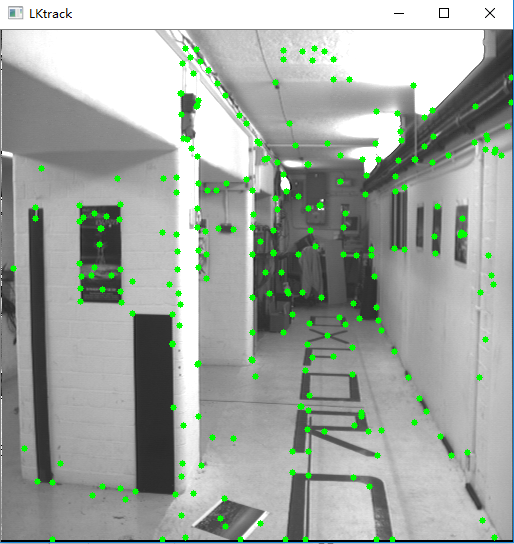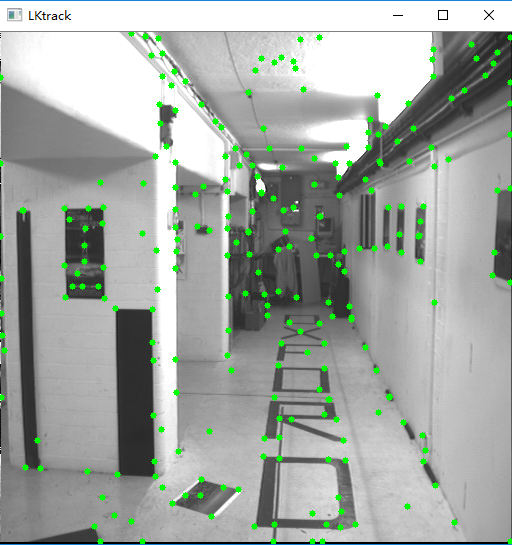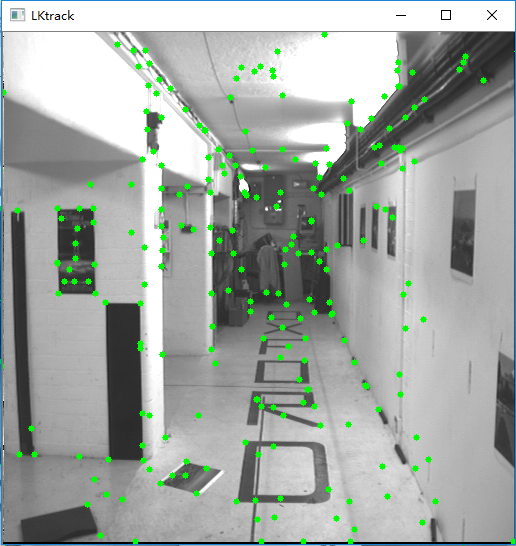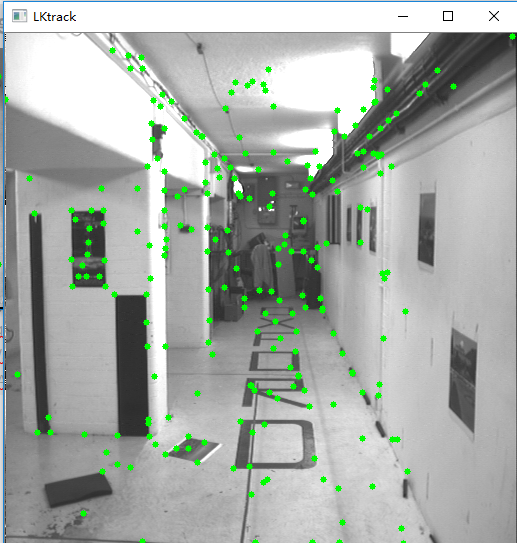2. 使用发生器

 def track(self):
"""  发生器，用于遍历整个序列 """

for i in range(len(self.imnames)):
if self.features == []:
self.detect_points()
else:
self.track_points()

#创建一份 RGB 副本
f = array(self.features).reshape(-1,2)
im = cv2.cvtColor(self.image,cv2.COLOR_BGR2RGB)
yield im,f
12345678910111213

# -*- coding: utf-8 -*-
import lktrack
import cv2
import numpy as np
from pylab import  *
from PIL import Image
imnames = ['F:\\Anaconda\\chapter10\\images (1)\\viff.000.ppm', 'F:\\Anaconda\\chapter10\\images (1)\\viff.001.ppm',
'F:\\Anaconda\\chapter10\\images (1)\\viff.002.ppm', 'F:\\Anaconda\\chapter10\\images (1)\\viff.003.ppm',
'F:\\Anaconda\\chapter10\\images (1)\\viff.004.ppm']
# 用 LKTracker 发生器进行跟踪
lkt = lktrack.LKTracker(imnames)
for im,ft in lkt.track():
print ('tracking %d features' % len(ft))
# 画出轨迹
figure()
imshow(im)
for p in ft:
plot(p,p,'bo')
for t in lkt.tracks:
plot([p for p in t],[p for p in t])
axis('off')
show()
12345678910111213141516171819202122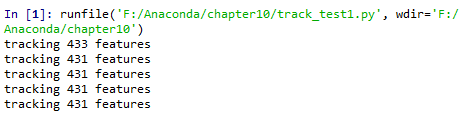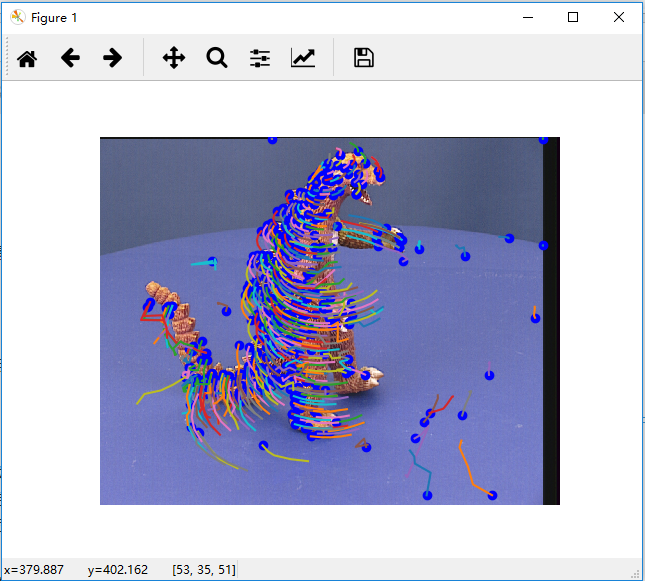imnames = ['F:\\Anaconda\\chapter10\\images\\bt.005.pgm','F:\\Anaconda\\chapter10\\images\\bt.004.pgm',
'F:\\Anaconda\\chapter10\\images\\bt.003.pgm', 'F:\\Anaconda\\chapter10\\images\\bt.002.pgm',
'F:\\Anaconda\\chapter10\\images\\bt.001.pgm', 'F:\\Anaconda\\chapter10\\images\\bt.000.pgm']
123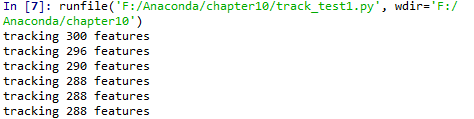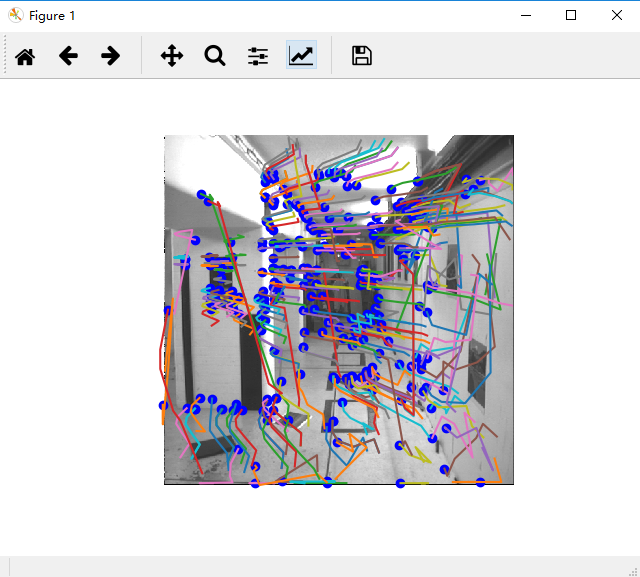# （五）更多示例

OpenCv自带很多关于如何使用python接口的有用示例。下面说明OpenCv的一些其他功能。

## （1）图像修复 cv2.inpaint()

OpenCv中的cv2.inpaint()函数可以实现图像修复的工作。图像修复的基本思路很简单：用邻近的像素替换那些坏标记，使其看起来像是邻居。

src： 输入8位1通道或3通道图像。
dst： 输出与src具有相同大小和类型的图像。
flags：

1. INPAINT_NS基于Navier-Stokes的方法
2. Alexandru Telea的INPAINT_TELEA方法

OpenCV提供了两种算法，两者都可以通过相同的函数访问，cv2.inpaint()

• 基于快速行进方法的图像修复技术：使用标志cv2.INPAINT_TELEA启用此算法
考虑图像中要修复的区域。算法从该区域的边界开始，然后进入区域内，逐渐填充边界中的所有内容。它需要在邻近的像素周围的一个小邻域进行修复。该像素由邻居中所有已知像素的归一化加权和代替。选择权重是一个重要的问题。对于靠近该点的那些像素，靠近边界的法线和位于边界轮廓上的像素，给予更多的权重。一旦像素被修复，它将使用快速行进方法移动到下一个最近的像素。FMM确保首先修复已知像素附近的像素，这样它就像手动启发式操作一样工作。

• 基于流体动力学的图像和视频修补：使用标志cv2.INPAINT_NS启用此算法
该算法基于流体动力学并利用偏微分方程。基本原则是heurisitic。它首先沿着已知区域的边缘行进到未知区域（因为边缘是连续的）。它继续等照片（连接具有相同强度的点的线，就像轮廓连接具有相同高度的点一样），同时在修复区域的边界处匹配渐变矢量。为此，使用来自流体动力学的一些方法。获得颜色后，填充颜色以减少该区域的最小差异。

# -*- coding: utf-8 -*-
import numpy as np
from matplotlib import pyplot as plt
import cv2

plt.subplot(221), plt.imshow(img)
plt.subplot(223), plt.imshow(dst_TELEA)
plt.title('TELEA')
plt.subplot(224), plt.imshow(dst_NS)
plt.title('NS')

plt.tight_layout()
plt.show()
12345678910111213141516171819202122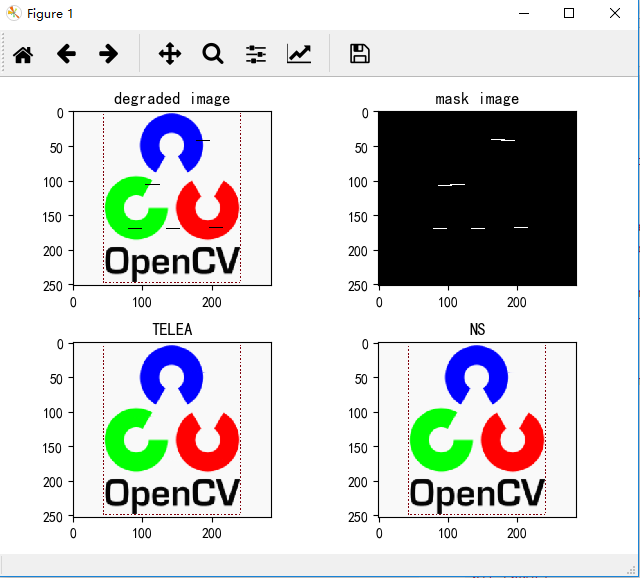## （2）利用分水岭变换进行分割 cv2.watershed()

1. 如果某个区域是前景或对象，就使用某个颜色（或灰度值）标签标记它。
2. 如果某个区域是背景就使用另外一个颜色标签标记。
3. 剩下的不能确定是前景还是背景的区域就用 0 标记。

# -*- coding: utf-8 -*-
import numpy as np
import cv2
from matplotlib import pyplot as plt

gray = cv2.cvtColor(img,cv2.COLOR_BGR2GRAY)
ret, thresh = cv2.threshold(gray,0,255,cv2.THRESH_BINARY_INV+cv2.THRESH_OTSU)

#cv2.imshow('ret',thresh)
#cv2.waitKey(0)
#cv2.destroyAllWindows()

# noise removal
kernel = np.ones((3,3),np.uint8)
opening = cv2.morphologyEx(thresh,cv2.MORPH_OPEN,kernel, iterations = 2) # 形态开运算

# sure background area
sure_bg = cv2.dilate(opening,kernel,iterations=3)

# Finding sure foreground area
dist_transform = cv2.distanceTransform(opening,cv2.DIST_L2,5)
ret, sure_fg = cv2.threshold(dist_transform,0.7*dist_transform.max(),255,0)

# Finding unknown region
sure_fg = np.uint8(sure_fg)
unknown = cv2.subtract(sure_bg,sure_fg)

# Marker labelling
ret, markers = cv2.connectedComponents(sure_fg)

# Add one to all labels so that sure background is not 0, but 1
markers = markers+1

# Now, mark the region of unknown with zero
markers[unknown==255] = 0

markers = cv2.watershed(img,markers)
img[markers == -1] = [255,0,0]

#cv2.imshow('img',img)
#cv2.waitKey(0)
#cv2.destroyAllWindows()

plt.subplot(421), plt.imshow(cv2.cvtColor(img, cv2.COLOR_BGR2RGB)),
plt.title('original'), plt.axis('off')
plt.subplot(422), plt.imshow(thresh, cmap='gray'),
plt.title('threshold'), plt.axis('off')
plt.subplot(423), plt.imshow(sure_bg, cmap='gray'),
plt.title('dilate'), plt.axis('off')
plt.subplot(424), plt.imshow(dist_transform, cmap='gray'),
plt.title('distanceTransform'), plt.axis('off')
plt.subplot(425), plt.imshow(sure_fg, cmap='gray'),
plt.title('threshold'), plt.axis('off')
plt.subplot(426), plt.imshow(unknown, cmap='gray'),
plt.title('subtract'), plt.axis('off')
plt.subplot(427), plt.imshow(np.abs(markers), cmap='jet'),
plt.title('mMarkers'), plt.axis('off')
plt.subplot(428), plt.imshow(cv2.cvtColor(img, cv2.COLOR_BGR2RGB)),
plt.title('result'), plt.axis('off')

plt.show()

12345678910111213141516171819202122232425262728293031323334353637383940414243444546474849505152535455565758596061626364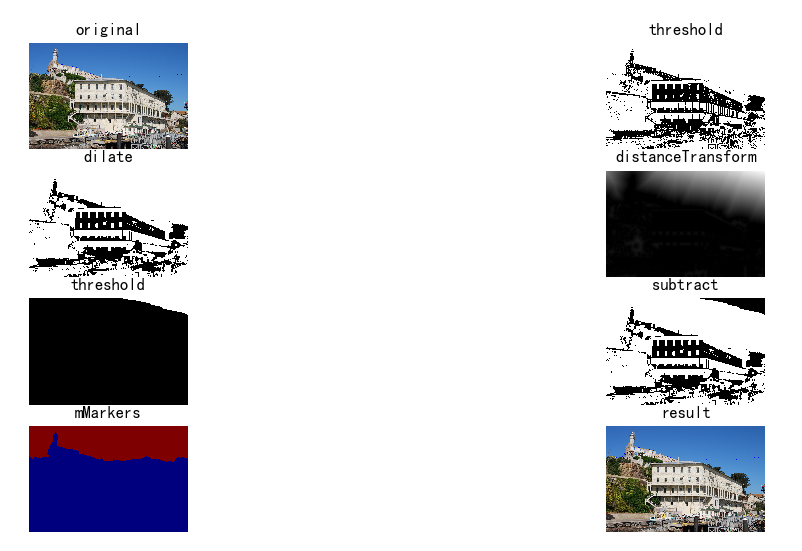## （3）利用霍夫变换检测直线 cv2.HoughLines()

OpenCV提供了两种用于直线检测的Hough变换形式，分别是cv2.HoughLinesP() 和cv2.HoughLines()。其中基本的版本是cv2.HoughLines。其输入一幅含有点集的二值图（由非0像素表示），其中一些点互相联系组成直线。

# -*- coding: utf-8 -*-
import cv2 as cv
import numpy as np

cv.imshow('img', img)

gray = cv.cvtColor(img, cv.COLOR_BGR2GRAY)
edges = cv.Canny(gray, 50, 150, apertureSize=3)
cv.imshow('edge', edges)

# 计算直线
lines = cv.HoughLines(edges, 1, np.pi / 180, 180)
tmp = img.copy()
for line in lines:
rho, theta = line
a = np.cos(theta)
b = np.sin(theta)
x0 = a * rho
y0 = b * rho
x1 = int(x0 + 1000 * (-b))
y1 = int(y0 + 1000 * (a))
x2 = int(x0 - 1000 * (-b))
y2 = int(y0 - 1000 * (a))
cv.line(tmp, (x1, y1), (x2, y2), (0, 0, 255), 4)
cv.imshow('line', tmp)

# 直接获取线段
lines = cv.HoughLinesP(edges, 1, np.pi / 180, 100, minLineLength=100, maxLineGap=10)
tmp = img.copy()
for line in lines:
x1, y1, x2, y2 = line
cv.line(tmp, (x1, y1), (x2, y2), (0, 0, 255), 4)
cv.imshow('line2', tmp)

cv.waitKey(0)
cv.destroyAllWindows()
1234567891011121314151617181920212223242526272829303132333435363738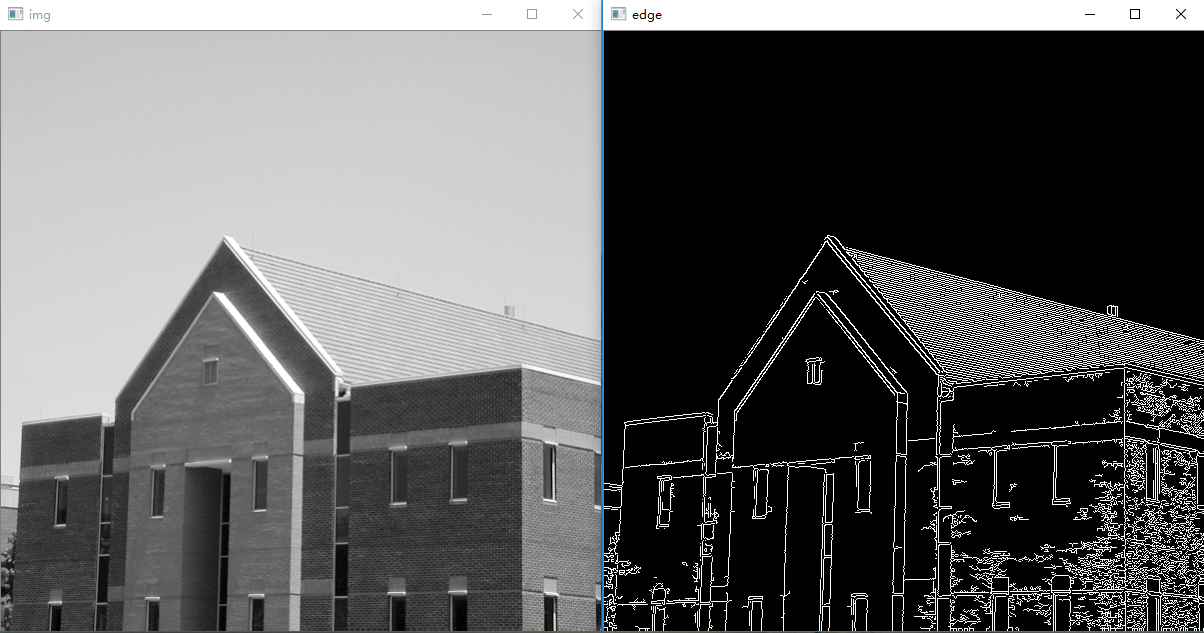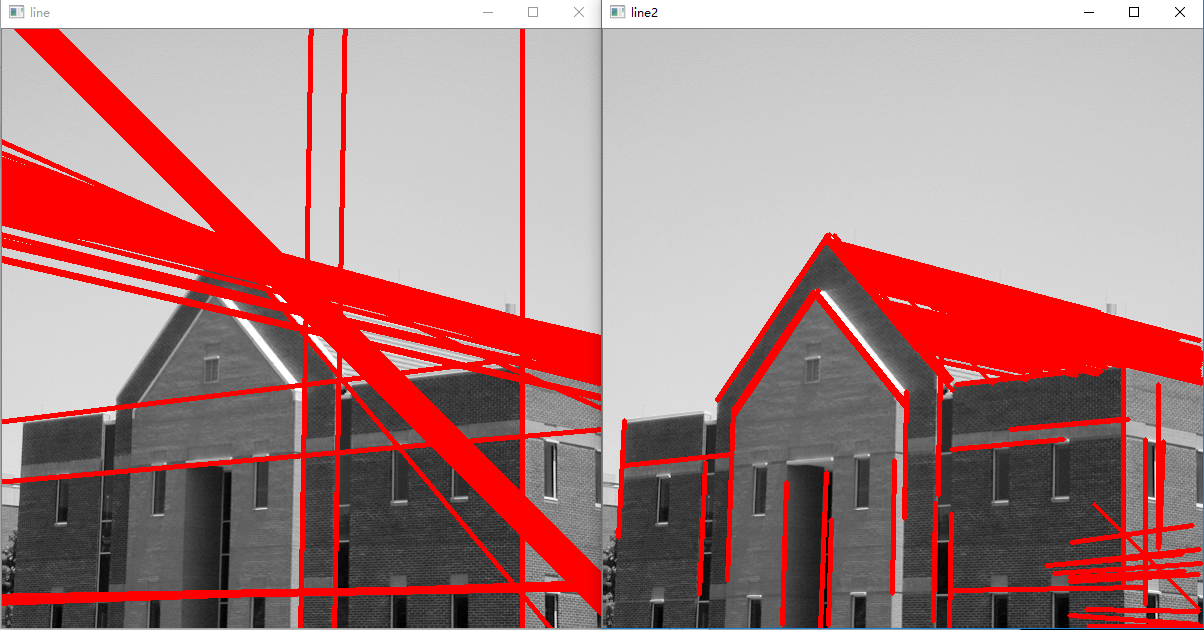20 0

pdf(new) 更多>